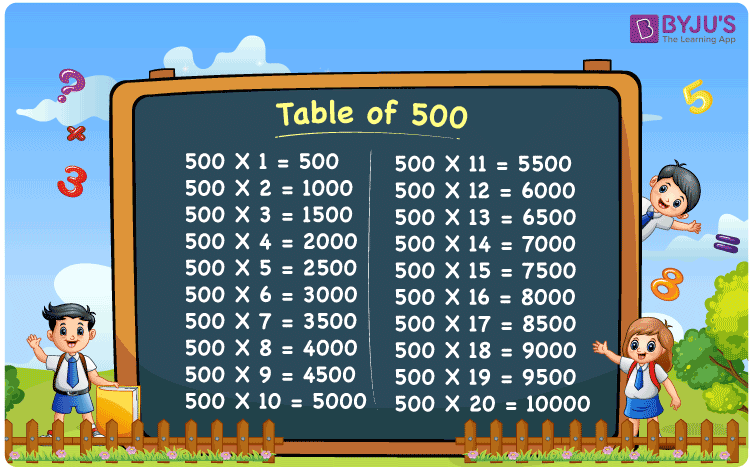Checkout JEE MAINS 2022 Question Paper Analysis : Checkout JEE MAINS 2022 Question Paper Analysis :

# Table of 500

The table of 500 is the multiplication table, which gives the multiples of 500. Learning a 500 times table is easy if you know the table of 5. In this article, we have provided the multiplication table of 500 in tabular form. Also, download the PDF for the table of 500 by clicking on the below link.

## Table of 500 Chart## What is the 500 Times Table?

The table that represents both multiplication and repeated addition of 500 is given below:

 500×1 = 500 500 500×2 = 1000 500 + 500 = 1000 500×3 = 1500 500 + 500 + 500 = 1500 500×4 = 2000 500 + 500 + 500 + 500 = 2000 500×5 = 2500 500 + 500 + 500 + 500 + 500 = 2500 500×6 = 3000 500 + 500 + 500 + 500 + 500 + 500 = 3000 500×7 = 3500 500 + 500 + 500 + 500 + 500 + 500 + 500 = 3500 500×8 = 4000 500 + 500 + 500 + 500 + 500 + 500 + 500 + 500 = 4000 500×9 = 4500 500 + 500 + 500 + 500 + 500 + 500 + 500 + 500 + 500 = 4500 500×10 = 5000 500 + 500 + 500 + 500 + 500 + 500 + 500 + 500 + 500 + 500 = 5000

## Multiplication Table of 500

The first 20 multiples of 500 are presented here in tabular form.

 500 × 1 = 500 500 × 2 = 1000 500 × 3 = 1500 500 × 4 = 2000 500 × 5 = 2500 500 × 6 = 3000 500 × 7 = 3500 500 × 8 = 4000 500 × 9 = 4500 500 × 10 = 5000 500 × 11 = 5500 500 × 12 = 6000 500 × 13 = 6500 500 × 14 = 7000 500 × 15 = 7500 500 × 16 = 8000 500 × 17 = 8500 500 × 18 = 9000 500 × 19 = 9500 500 × 20 = 10000

## Solved Example on the Table of 500

Example:

What is 500 more than 10 times 500?

Solution:

We know that 10 times 500 is 5000.

I.e., 10 × 500 = 5000.

Therefore, 500 more than the 10 times 500 = 500 + 5000 = 5500.

## Frequently Asked Questions on the Table of 500

### What is the table of 500?

The table of 500 is the 500 times table which gives the multiples of 500.

### How many times does 500 equal 8000?

16 times 500 equals 8000.

### What is 500 times 20?

500 times 20 is 10000. I.e., 500 × 20 = 10000.